# C++ Programming Examples

Since there are an extensive number of programs that can be created using C++. Therefore, we have created a series of C++ programming examples that are divided into more than 100 articles. In each article, you will get one or more programs with well-explained code and its output.

We've listed more than 500 C++ programs along with their outputs, from the simplest one to shutting down your computer using a C++ program.

From the list of all programs, here is a list of some popular programs in C++:

Note: Each and every program in C++ given here has been well tested and executed. You can check it out for yourself by visiting some of the programs given above.

But before starting the series of C++ programming examples, Let's first go through some of the interesting programs given in this article. Let's start with the simplest C++ program, as shown in the following example.

## C++ Program Example 1

Here is the simplest C++ program that will print the string, "Hello Compiler, I am C++," on the output. Let's have a look at the program given below:

```// C++ Programming Example No.1

#include<iostream>
using namespace std;
int main()
{
cout<<"Hello Compiler, I am C++";
cout<<endl;
return 0;
}```

This program was built and runs under the Code::Blocks IDE. Here is its sample output: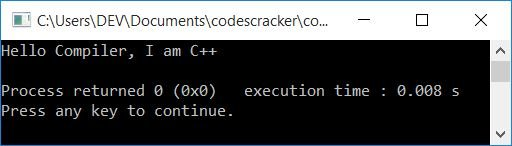The iostream is a header file that stands for input/output stream and provides basic input/output services for the C++ program. Like in the above program, cout is used to put some content on the output screen. It is defined in the iostream header file.

The following C++ statement:

`using namespace std;`

is used to provide standard (std) input and output namespaces. That is, after including this statement, we do not need to write std:: before every cout and cin.

endl stands for "end of line", and it is used to break the line and begin the next thing on a new line.

## C++ Program Example 2

Here is the second example in C++ programming.

```// C++ Programming Example No.2

#include<iostream>
using namespace std;
int main()
{
char str;
cin>>str;
cout<<"\nHello "<<str<<" Sir, This is codescracker.com!";
cout<<endl;
return 0;
}```

This is the initial output produced by the above program: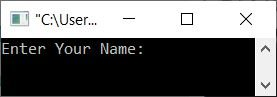Now supply your name, say Albert, and press the ENTER key to see the following output: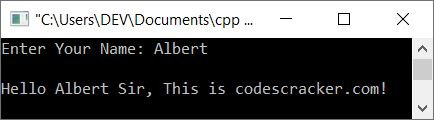## C++ Program Example 3

Let's take a look at the third example of C++ given below. This program receives a number as input from the user and checks whether it is greater than 10 and less than 100 or not to print a message accordingly.

```// C++ Programming Example No.3

#include<iostream>
using namespace std;
int main()
{
int num;
cout<<"Gues a Number: ";
cin>>num;
if(num>10 && num<100)
cout<<"\nWhat a True Guess!";
else
cout<<"\nOpps!";
cout<<endl;
return 0;
}```

Here is its sample run with user input: 14.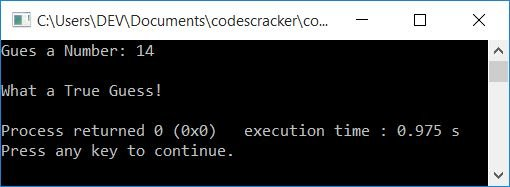And here is another sample run with user input: 3.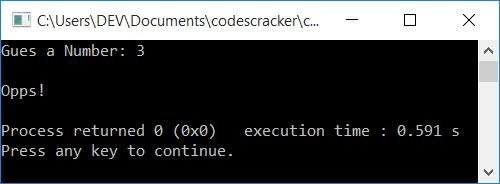## C++ Program Example 4

This is the fourth example program in C++. This program asks the user to create a password and then receives input as two numbers. However, before adding these two numbers and printing the result, the program prompts you to enter the password you created earlier (in the same program):

If the user enters the correct password, then the program calculates and displays the result; otherwise, it doesn't display the result, but rather shows a message like "Wrong Password!"

```// C++ Programming Example No. 4

#include<iostream>
#include<string.h>
using namespace std;
int main()
{
char pass, ePass;
int numOne, numTwo, sum;
cin>>pass;
cout<<"\nEnter Two Numbers to Add: ";
cin>>numOne>>numTwo;
cout<<"\nEnter the Password to See the Result: ";
cin>>ePass;
if(!strcmp(pass, ePass))
{
sum = numOne + numTwo;
cout<<endl<<numOne<<" + "<<numTwo<<" = "<<sum;
}
else
cout<<"\nSorry! You've entered a Wrong Password!";
cout<<endl;
return 0;
}```

Here is a sample run of this C++ program. Below is the initial output:Now supply the following inputs:

• To create a new password, use codescracker.
• 40 and 60 as two numbers
• codescracker as a password to see the sum of two numbers entered

Here is the output after providing these inputs: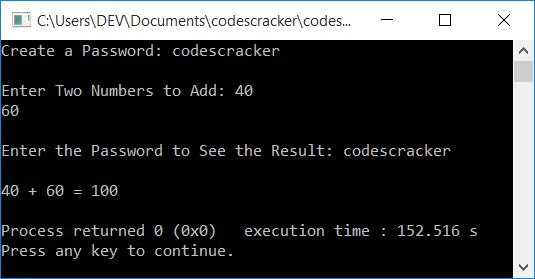If you enter the wrong password, then you will not see the result; rather, the output produced by the above program is "Sorry! You've entered the wrong password!".

## C++ Program Example 5

This is the fifth example program in C++. This program confirms whether the user is a robot or not.

```// C++ Programming Example No. 5

#include<iostream>
#include<string.h>
using namespace std;
int main()
{
char robotChk;
int val;
cout<<"Are You a Robot ? ";
cin>>robotChk;
val = strcmp("yes", robotChk);
if(val==0)
cout<<"\nYou can't Proceed!";
else
cout<<"\nYou're Welcome!";
cout<<endl;
return 0;
}```

Here is the sample run of the above C++ program with user input, yes: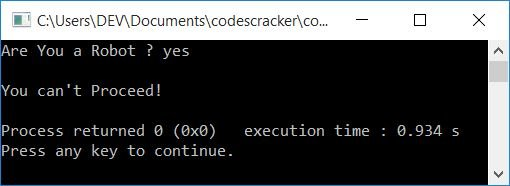And here is another sample run with user input, no: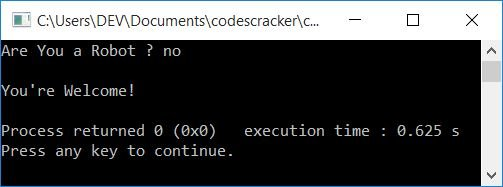## C++ Program Example 6

This is the last (sixth) example program in C++ that asks to enter the name of the user to check whether he or she is invited or not.

```// C++ Programming Example No.6

#include<iostream>
#include<string.h>
using namespace std;
int main()
{
char arr = {"programmer", "developer", "engineer",
"student", "teacher", "professor"};
char name;
int found=0;
cout<<"Who are You ? ";
cin>>name;
for(int i=0; i<6; i++)
{
if(!strcmp(name, arr[i]))
{
cout<<"\nCongratulation!";
cout<<"\nYou're invited from codescracker.com";
found=1;
break;
}
else
found++;
}
if(found != 1)
cout<<"\nSorry!\nYou're not invited";
cout<<endl;
return 0;
}```

Here is its sample run with user input, programmer: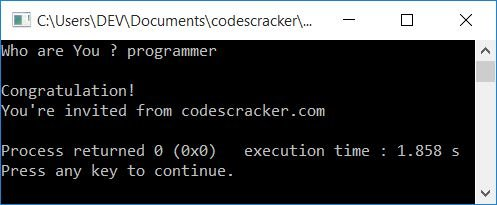Note: Don't panic if you're not getting an idea of what is going on behind the C++ codes given above. Because this is just a program that shows you how it looks. Starting with a very simple program, printing "Hello, world," to an advanced level program, you will learn about all of these things one by one in the following program (article).

### For TurboC++ User

Because all programs given here are well-tested and compiled through the Code::Blocks IDE, Therefore, if you are a TurboC++ user, it is better to skip it and continue with the IDE used here. But if you don't, then do these things:

• Include .h after every header file's name. For example, replace iostream with iostream.h. If the header file already contains.h, do not make the change.
• Remove the statement, using namespace std;
• Change int main() to void main().
• getch() should be used instead of return 0.

#### Other Languages Programming Examples

You may also like to go through and learn about all these programs in other languages, such as:

C++ Online Test

« C++ Tutorial Next Program »